# ML Aggarwal Solutions for Class 9 Maths Chapter 7 - Quadratic Equations

ML Aggarwal Solutions for Class 9 Maths Chapter 7 – Quadratic Equations are provided here to help students understand all the concepts clearly and develop a strong command over the subject. This chapter mainly deals with problems based on quadratic equations. Sometimes, students face difficulty in understanding the concepts during class hours, for those students, the experts at BYJU’S have designed the solutions based on the students’ grasping abilities. Students can solve both chapter-wise and exercise wise problems to increase their confidence level before appearing for the board exam. To boost interest among students in this chapter, ML Aggarwal Solutions for Class 9 Chapter 7 quadratic equations pdf links are given here for easy access. Students can download the pdfs easily and can use it for future reference as well.

Chapter 7 – Quadratic Equations contains one exercise and the

ML Aggarwal Class 9 Solutions present in this page provide solutions to questions related to each topic discussed in this chapter.

## Download the Pdf of ML Aggarwal Solutions for Class 9 Maths Chapter 7 – Quadratic Equations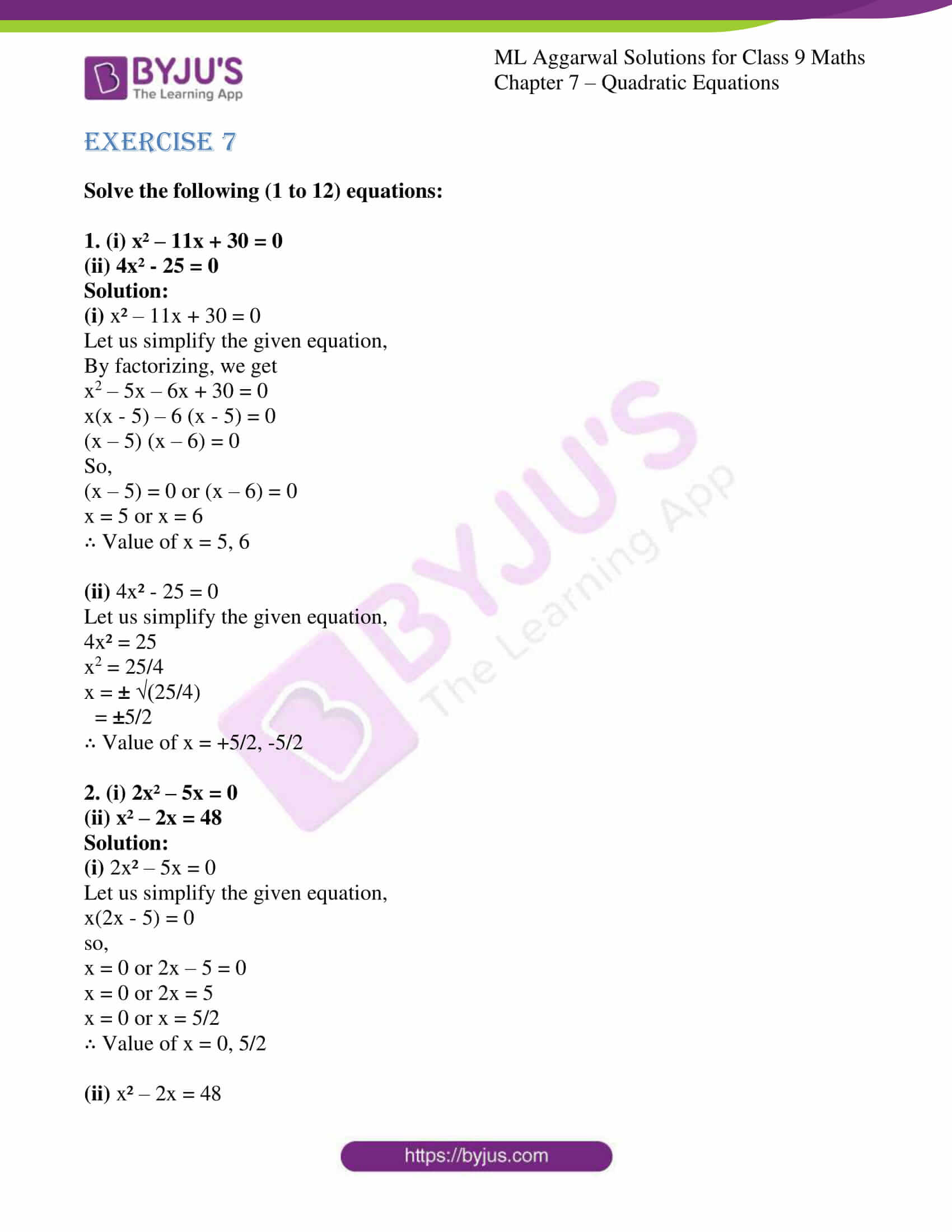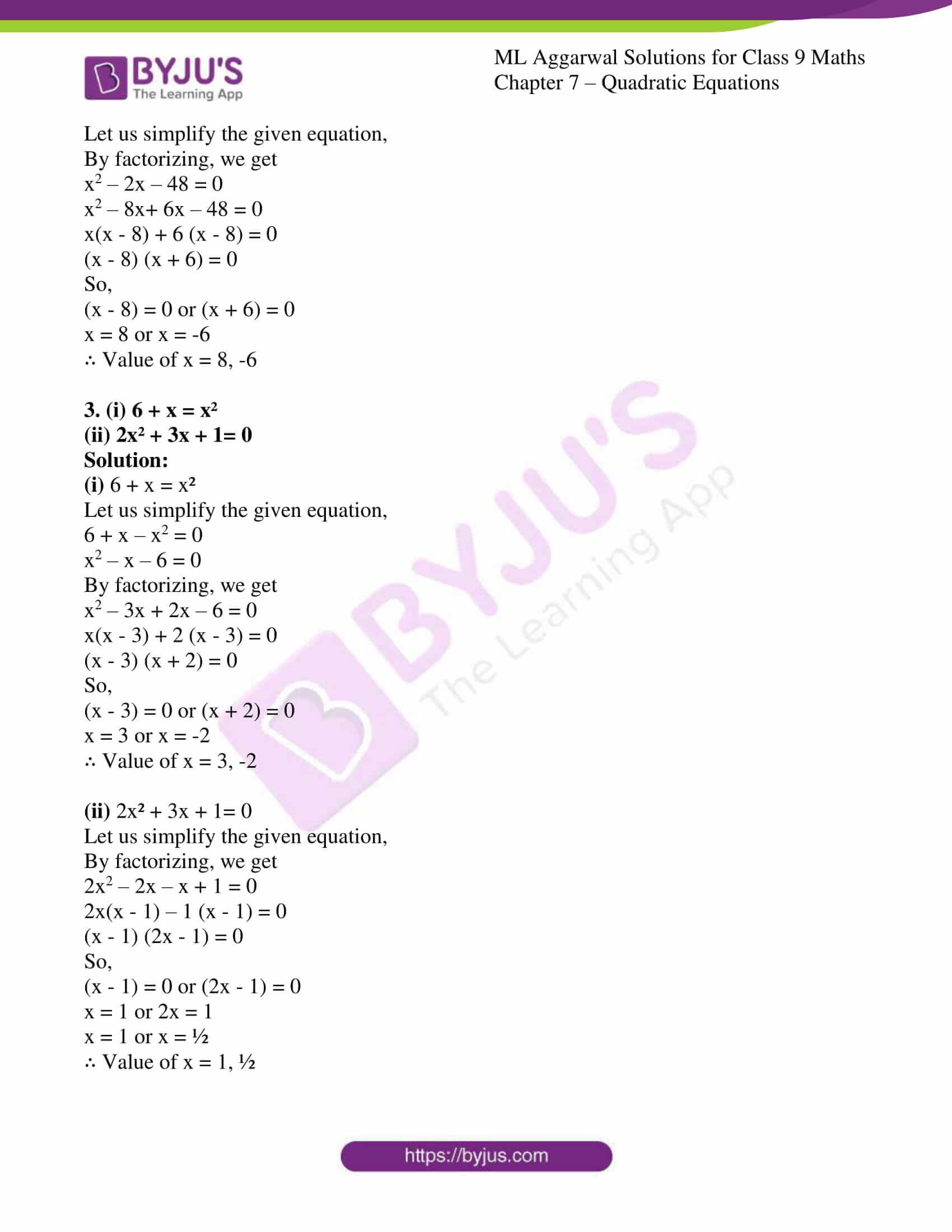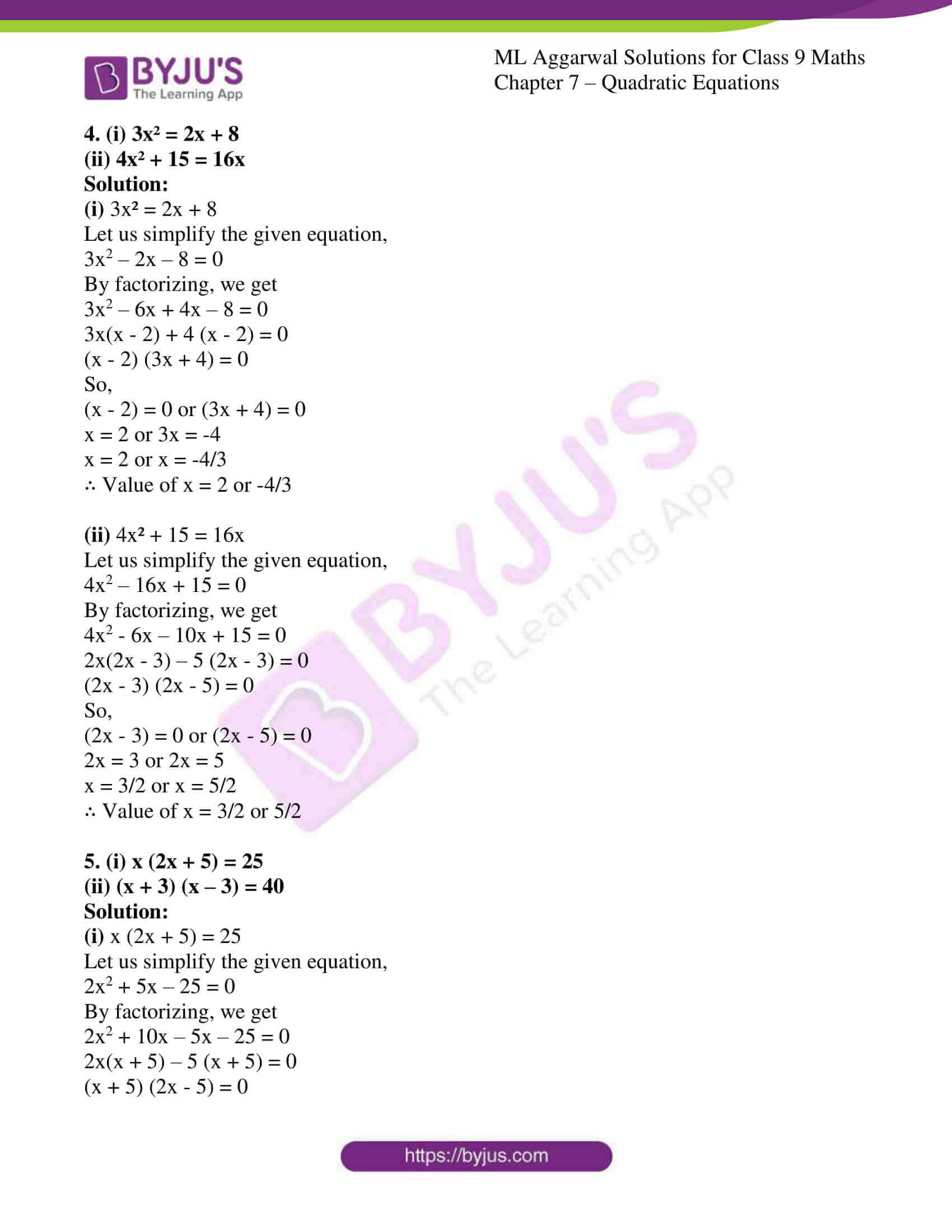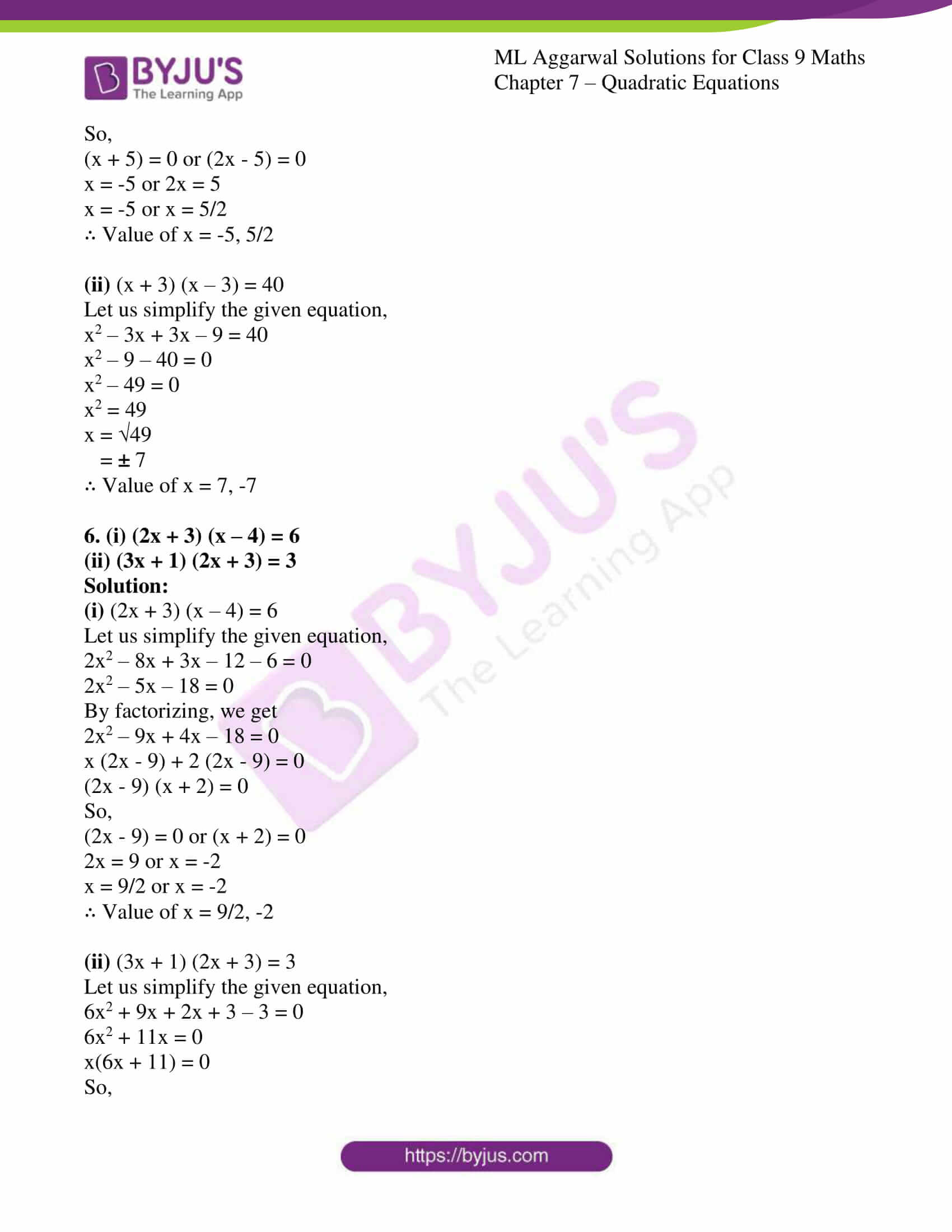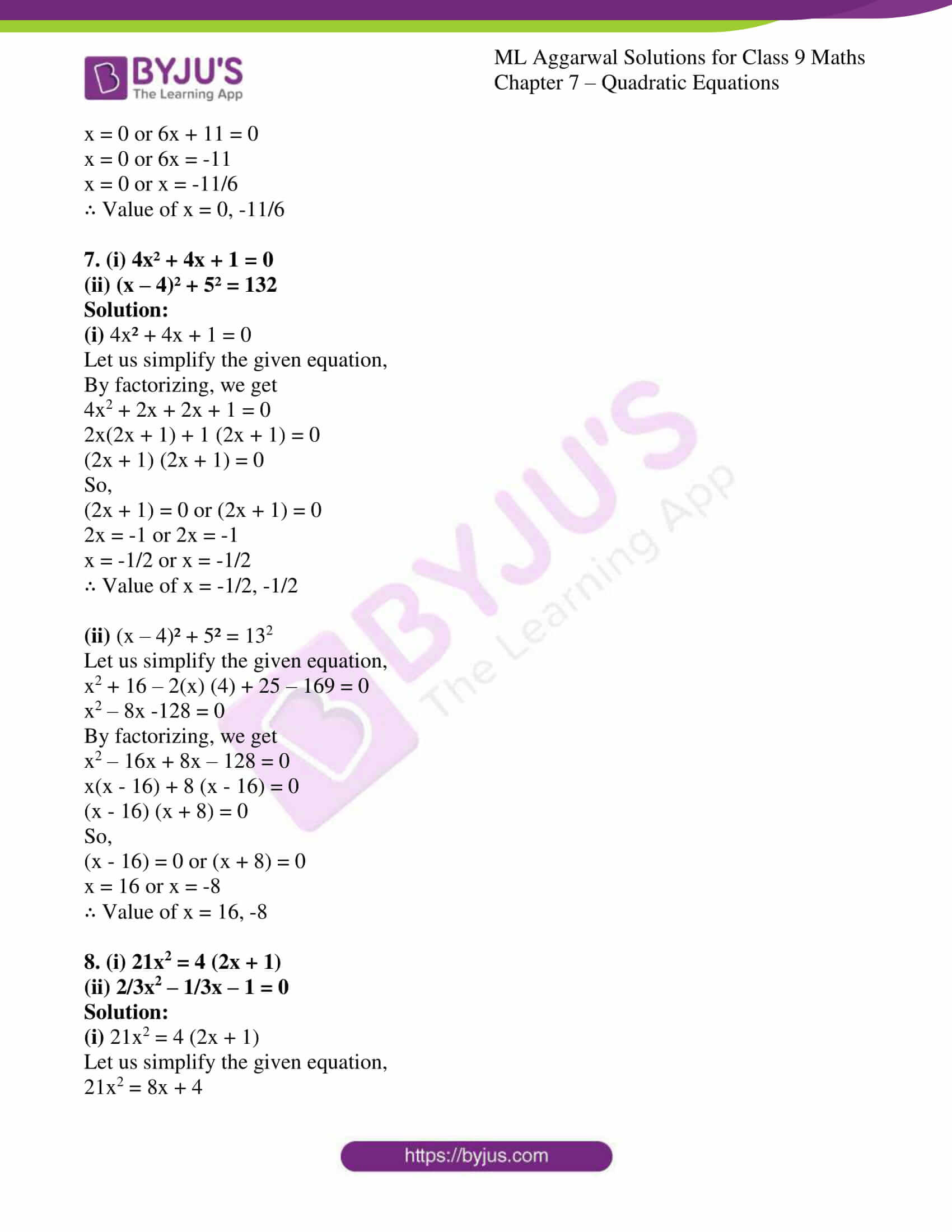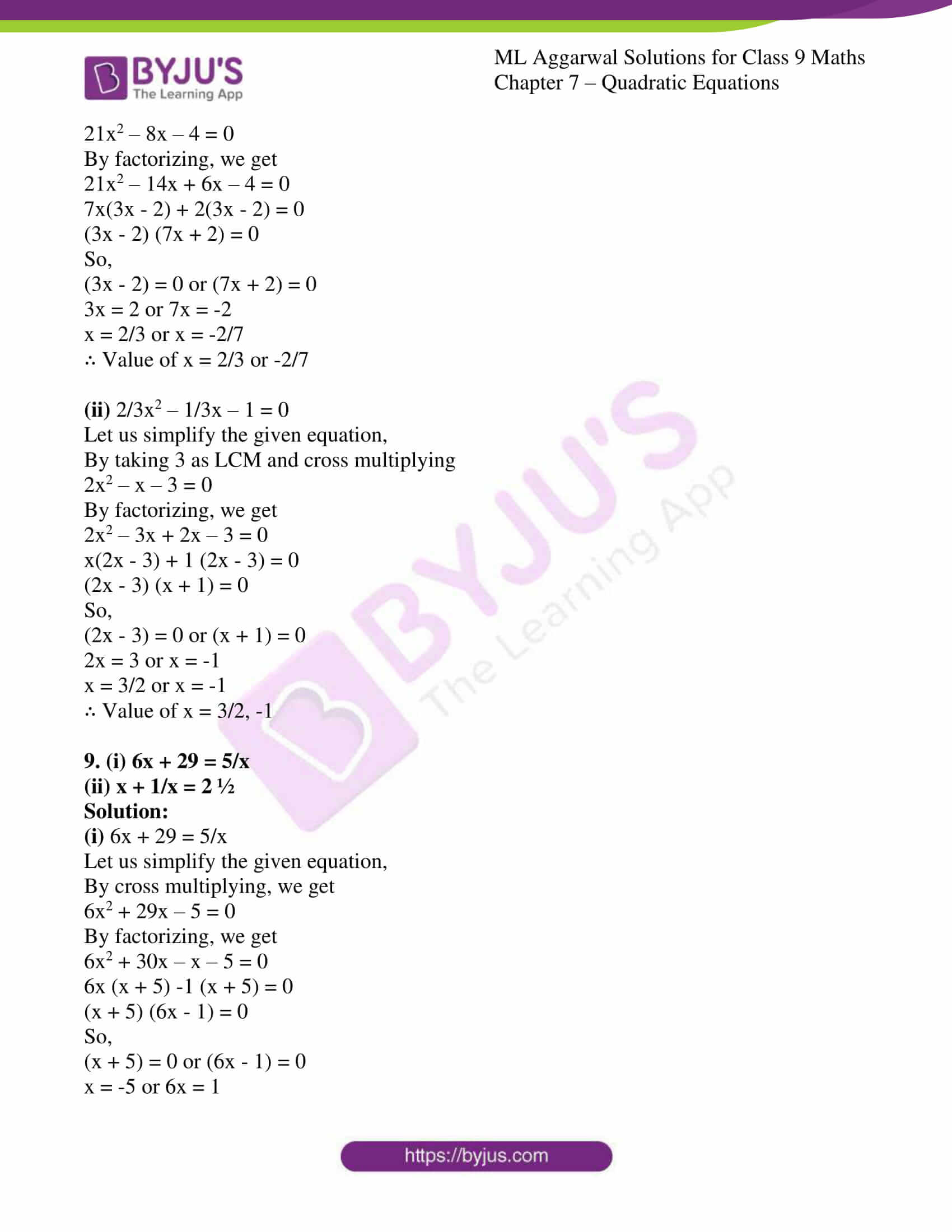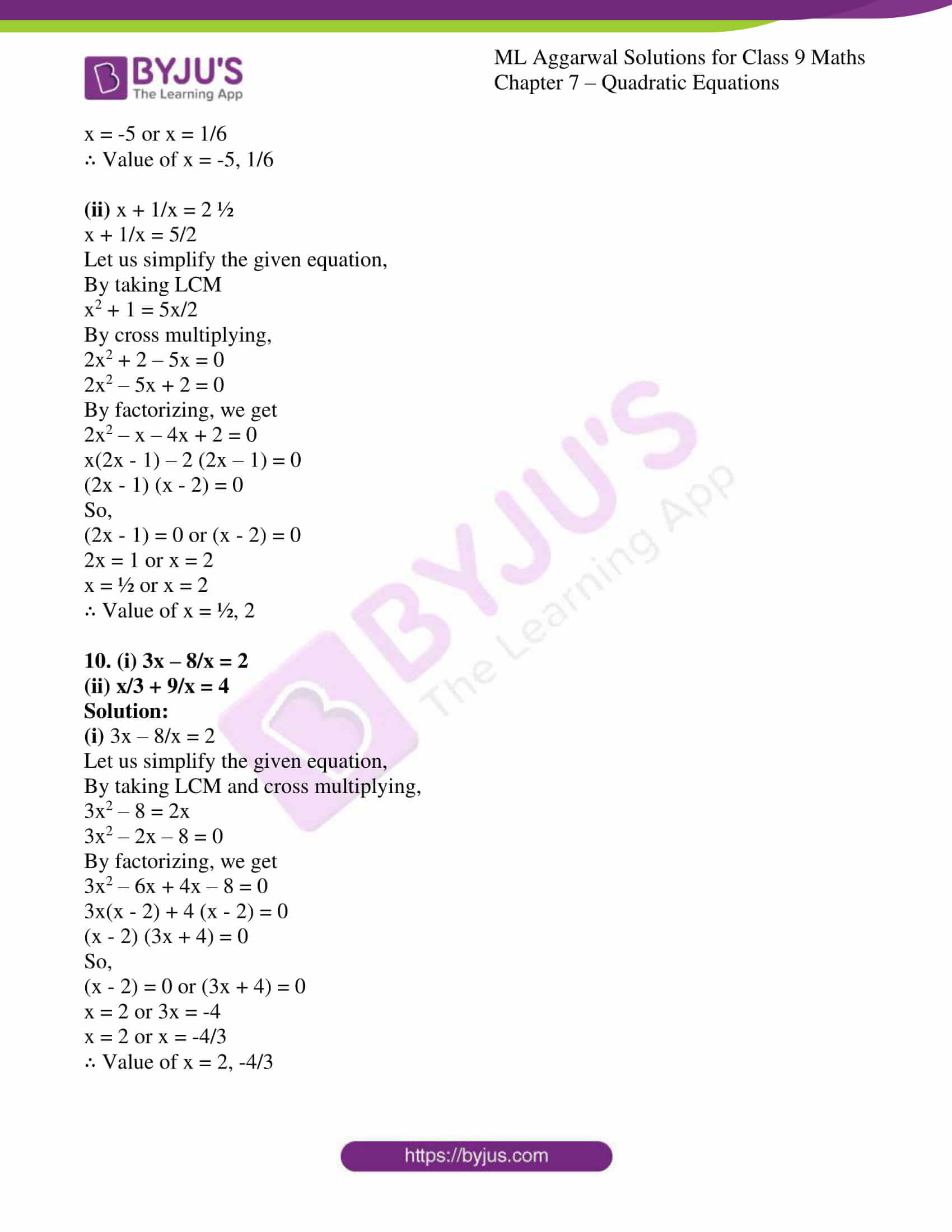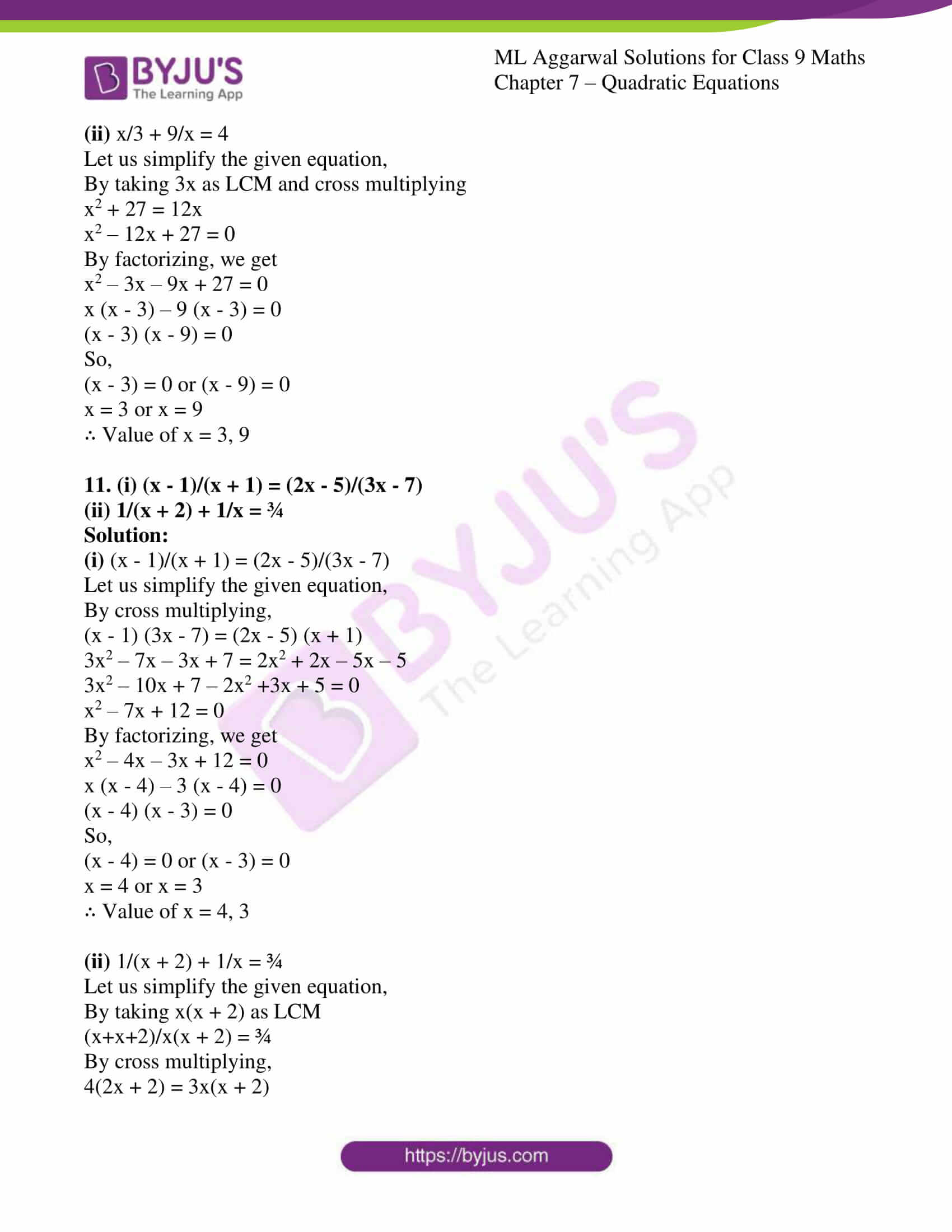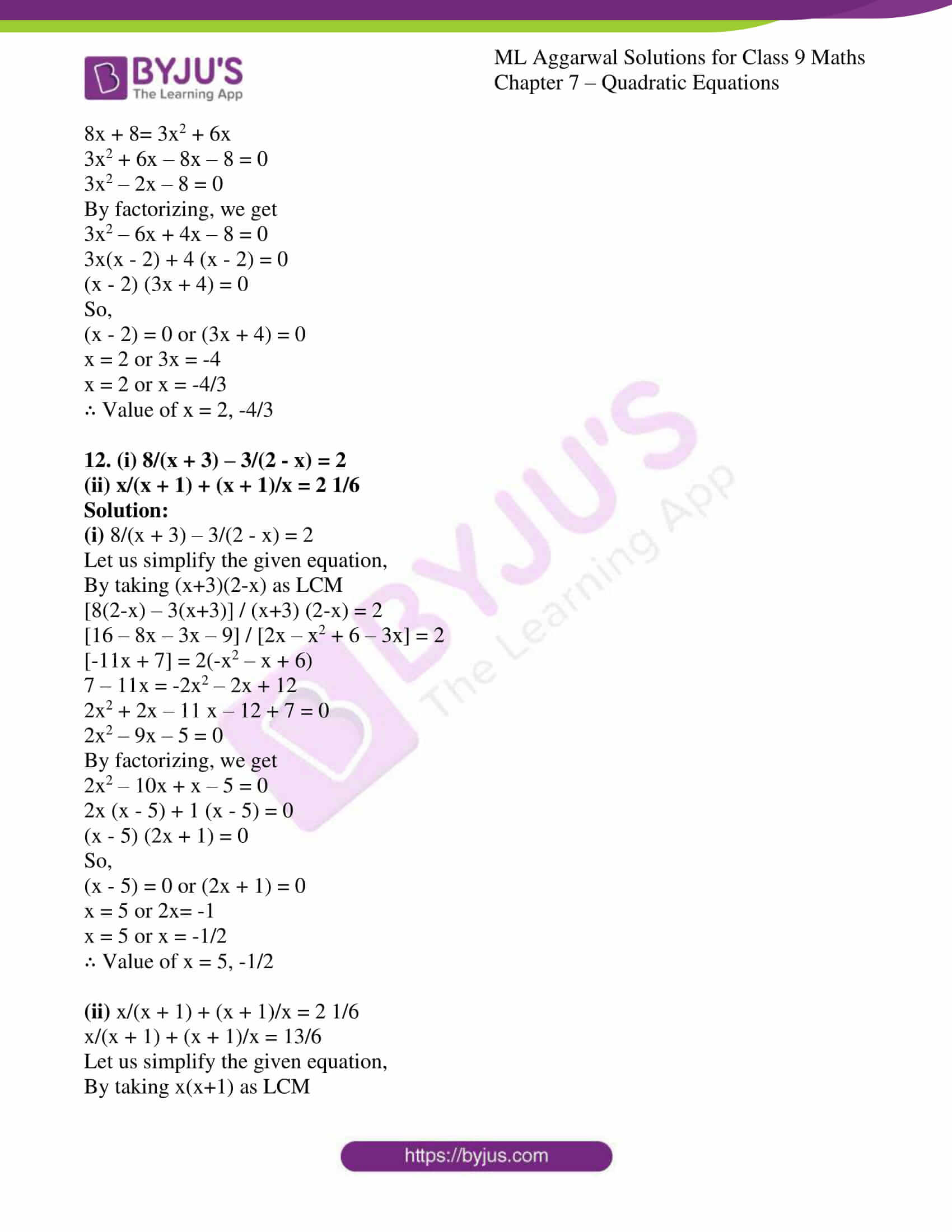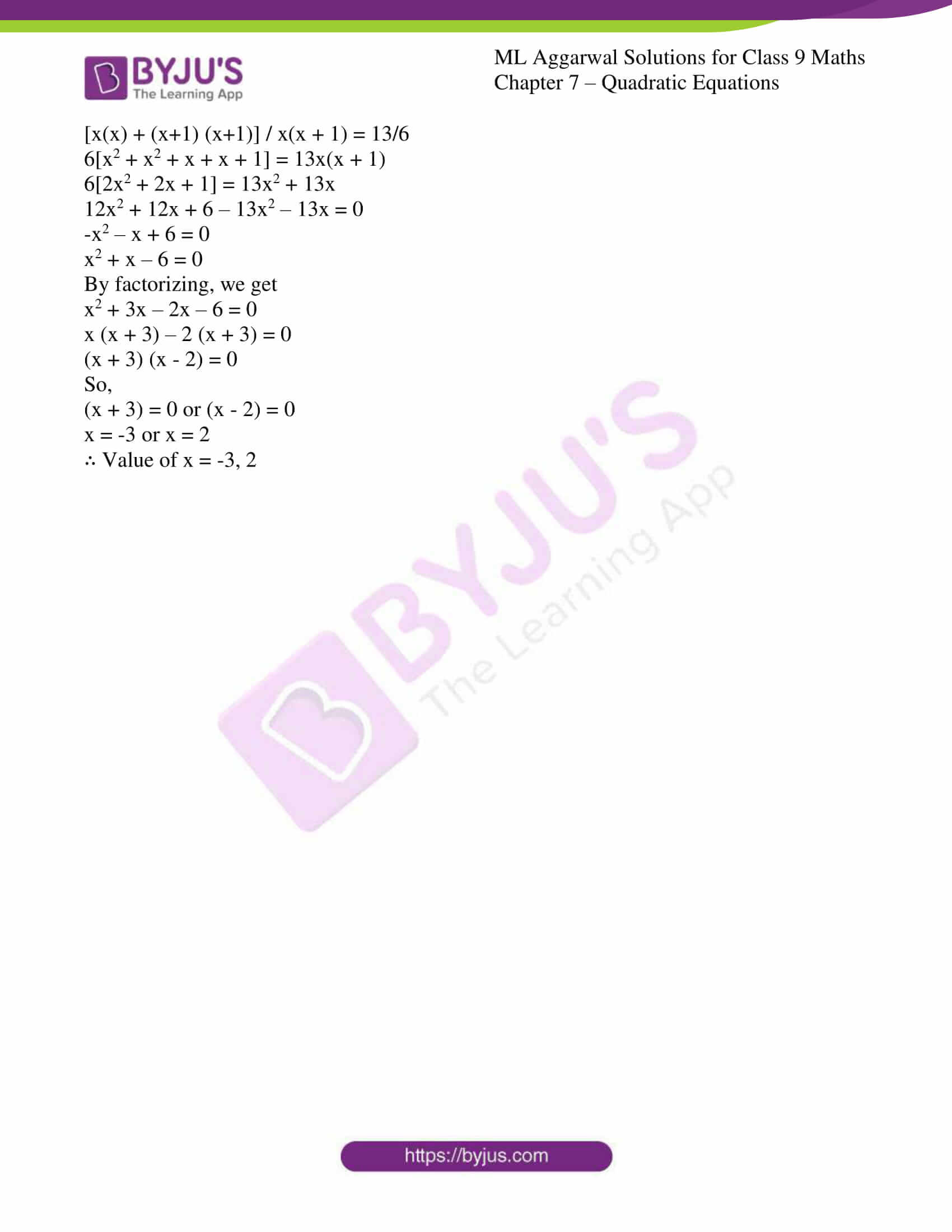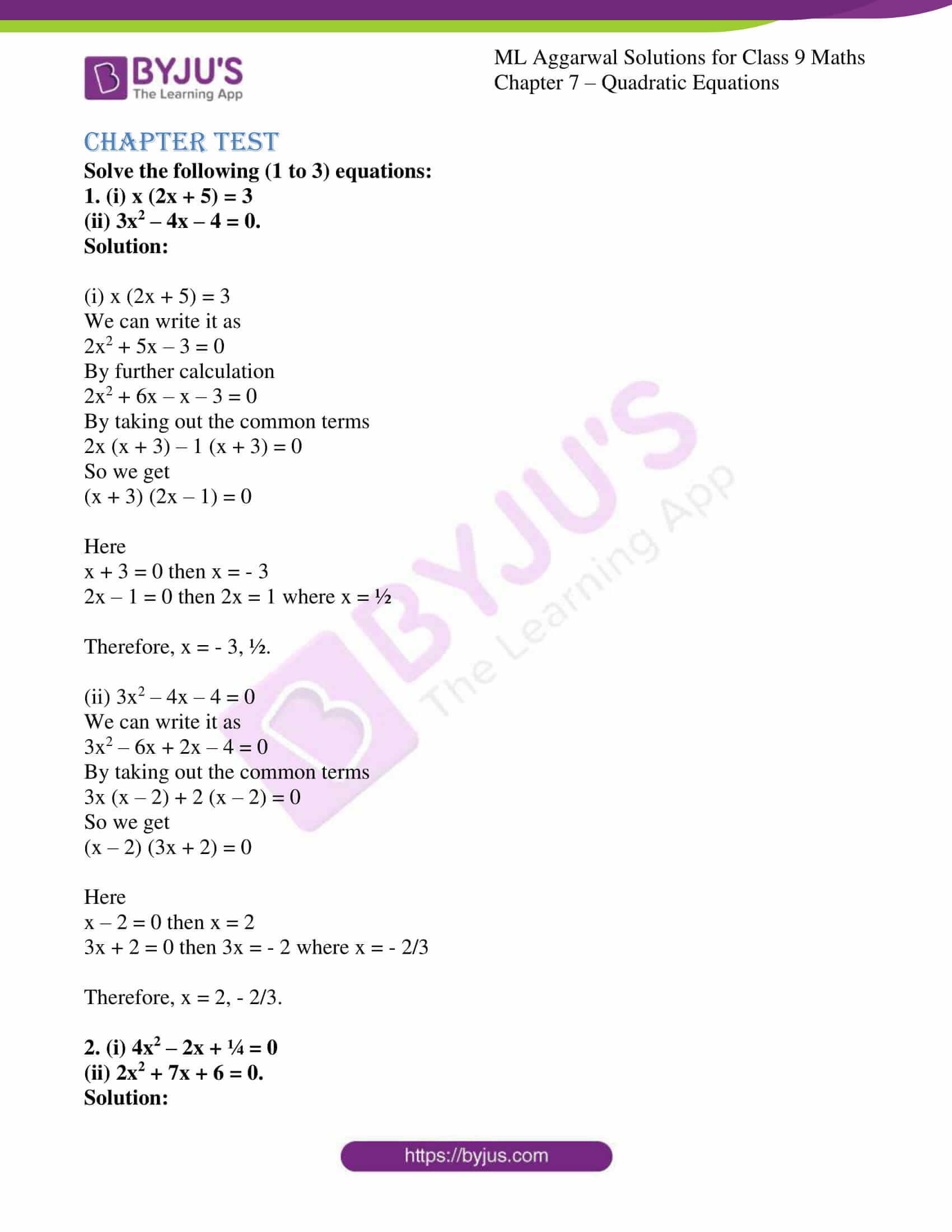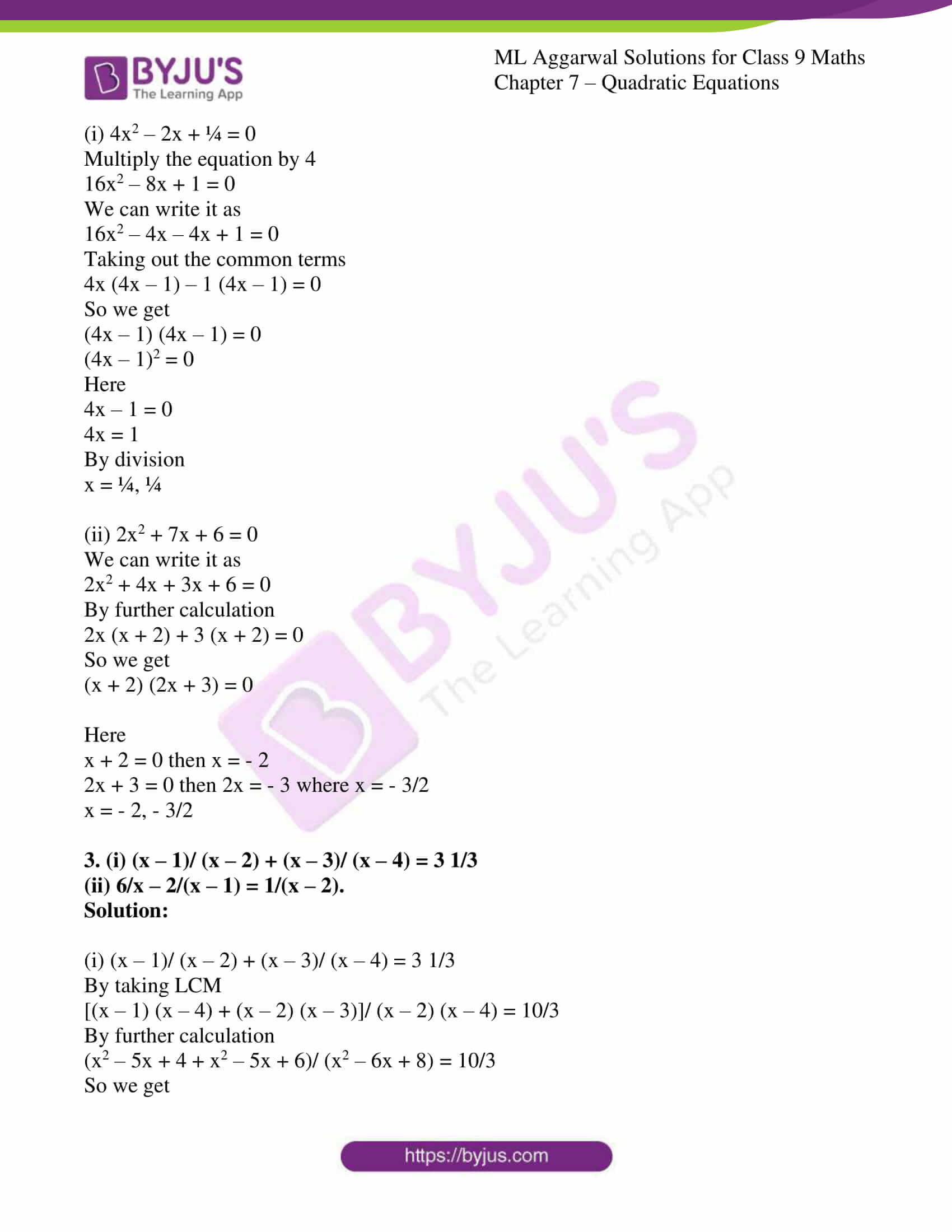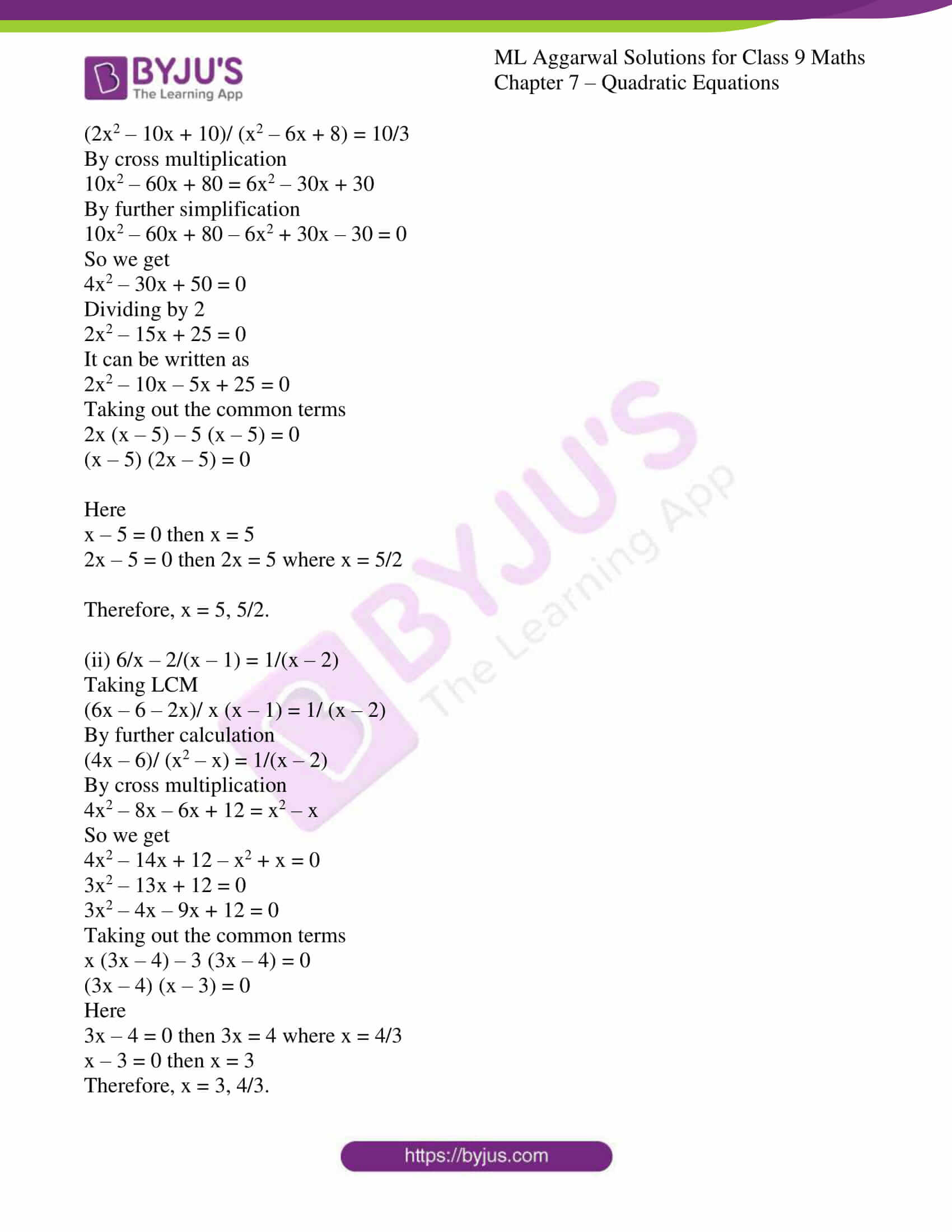### Access answers to ML Aggarwal Solutions for Class 9 Maths Chapter 7 – Quadratic Equations

EXERCISE 7

Solve the following (1 to 12) equations:

1. (i) x² – 11x + 30 = 0
(ii) 4x² – 25 = 0

Solution:

(i) x² – 11x + 30 = 0

Let us simplify the given equation,

By factorizing, we get

x2 – 5x – 6x + 30 = 0

x(x – 5) – 6 (x – 5) = 0

(x – 5) (x – 6) = 0

So,

(x – 5) = 0 or (x – 6) = 0

x = 5 or x = 6

∴ Value of x = 5, 6

(ii) 4x² – 25 = 0

Let us simplify the given equation,

4x² = 25

x2 = 25/4

x = ± √(25/4)

= ±5/2

∴ Value of x = +5/2, -5/2

2. (i) 2x² – 5x = 0

(ii) x² – 2x = 48

Solution:

(i) 2x² – 5x = 0

Let us simplify the given equation,

x(2x – 5) = 0

so,

x = 0 or 2x – 5 = 0

x = 0 or 2x = 5

x = 0 or x = 5/2

∴ Value of x = 0, 5/2

(ii) x² – 2x = 48

Let us simplify the given equation,

By factorizing, we get

x2 – 2x – 48 = 0

x2 – 8x+ 6x – 48 = 0

x(x – 8) + 6 (x – 8) = 0

(x – 8) (x + 6) = 0

So,

(x – 8) = 0 or (x + 6) = 0

x = 8 or x = -6

∴ Value of x = 8, -6

3. (i) 6 + x = x²

(ii) 2x² + 3x + 1= 0

Solution:

(i) 6 + x = x²

Let us simplify the given equation,

6 + x – x2 = 0

x2 – x – 6 = 0

By factorizing, we get

x2 – 3x + 2x – 6 = 0

x(x – 3) + 2 (x – 3) = 0

(x – 3) (x + 2) = 0

So,

(x – 3) = 0 or (x + 2) = 0

x = 3 or x = -2

∴ Value of x = 3, -2

(ii) 2x² + 3x + 1= 0

Let us simplify the given equation,

By factorizing, we get

2x2 – 2x – x + 1 = 0

2x(x – 1) – 1 (x – 1) = 0

(x – 1) (2x – 1) = 0

So,

(x – 1) = 0 or (2x – 1) = 0

x = 1 or 2x = 1

x = 1 or x = ½

∴ Value of x = 1, ½

4. (i) 3x² = 2x + 8
(ii) 4x² + 15 = 16x

Solution:

(i) 3x² = 2x + 8

Let us simplify the given equation,

3x2 – 2x – 8 = 0

By factorizing, we get

3x2 – 6x + 4x – 8 = 0

3x(x – 2) + 4 (x – 2) = 0

(x – 2) (3x + 4) = 0

So,

(x – 2) = 0 or (3x + 4) = 0

x = 2 or 3x = -4

x = 2 or x = -4/3

∴ Value of x = 2 or -4/3

(ii) 4x² + 15 = 16x

Let us simplify the given equation,

4x2 – 16x + 15 = 0

By factorizing, we get

4x2 – 6x – 10x + 15 = 0

2x(2x – 3) – 5 (2x – 3) = 0

(2x – 3) (2x – 5) = 0

So,

(2x – 3) = 0 or (2x – 5) = 0

2x = 3 or 2x = 5

x = 3/2 or x = 5/2

∴ Value of x = 3/2 or 5/2

5. (i) x (2x + 5) = 25

(ii) (x + 3) (x – 3) = 40

Solution:

(i) x (2x + 5) = 25

Let us simplify the given equation,

2x2 + 5x – 25 = 0

By factorizing, we get

2x2 + 10x – 5x – 25 = 0

2x(x + 5) – 5 (x + 5) = 0

(x + 5) (2x – 5) = 0

So,

(x + 5) = 0 or (2x – 5) = 0

x = -5 or 2x = 5

x = -5 or x = 5/2

∴ Value of x = -5, 5/2

(ii) (x + 3) (x – 3) = 40

Let us simplify the given equation,

x2 – 3x + 3x – 9 = 40

x2 – 9 – 40 = 0

x2 – 49 = 0

x2 = 49

x = √49

= ± 7

∴ Value of x = 7, -7

6. (i) (2x + 3) (x – 4) = 6
(ii) (3x + 1) (2x + 3) = 3

Solution:

(i) (2x + 3) (x – 4) = 6

Let us simplify the given equation,

2x2 – 8x + 3x – 12 – 6 = 0

2x2 – 5x – 18 = 0

By factorizing, we get

2x2 – 9x + 4x – 18 = 0

x (2x – 9) + 2 (2x – 9) = 0

(2x – 9) (x + 2) = 0

So,

(2x – 9) = 0 or (x + 2) = 0

2x = 9 or x = -2

x = 9/2 or x = -2

∴ Value of x = 9/2, -2

(ii) (3x + 1) (2x + 3) = 3

Let us simplify the given equation,

6x2 + 9x + 2x + 3 – 3 = 0

6x2 + 11x = 0

x(6x + 11) = 0

So,

x = 0 or 6x + 11 = 0

x = 0 or 6x = -11

x = 0 or x = -11/6

∴ Value of x = 0, -11/6

7. (i) 4x² + 4x + 1 = 0
(ii) (x – 4)² + 5² = 132

Solution:

(i) 4x² + 4x + 1 = 0

Let us simplify the given equation,

By factorizing, we get

4x2 + 2x + 2x + 1 = 0

2x(2x + 1) + 1 (2x + 1) = 0

(2x + 1) (2x + 1) = 0

So,

(2x + 1) = 0 or (2x + 1) = 0

2x = -1 or 2x = -1

x = -1/2 or x = -1/2

∴ Value of x = -1/2, -1/2

(ii) (x – 4)² + 5² = 132

Let us simplify the given equation,

x2 + 16 – 2(x) (4) + 25 – 169 = 0

x2 – 8x -128 = 0

By factorizing, we get

x2 – 16x + 8x – 128 = 0

x(x – 16) + 8 (x – 16) = 0

(x – 16) (x + 8) = 0

So,

(x – 16) = 0 or (x + 8) = 0

x = 16 or x = -8

∴ Value of x = 16, -8

8. (i) 21x2 = 4 (2x + 1)

(ii) 2/3x2 – 1/3x – 1 = 0

Solution:

(i) 21x2 = 4 (2x + 1)

Let us simplify the given equation,

21x2 = 8x + 4

21x2 – 8x – 4 = 0

By factorizing, we get

21x2 – 14x + 6x – 4 = 0

7x(3x – 2) + 2(3x – 2) = 0

(3x – 2) (7x + 2) = 0

So,

(3x – 2) = 0 or (7x + 2) = 0

3x = 2 or 7x = -2

x = 2/3 or x = -2/7

∴ Value of x = 2/3 or -2/7

(ii) 2/3x2 – 1/3x – 1 = 0

Let us simplify the given equation,

By taking 3 as LCM and cross multiplying

2x2 – x – 3 = 0

By factorizing, we get

2x2 – 3x + 2x – 3 = 0

x(2x – 3) + 1 (2x – 3) = 0

(2x – 3) (x + 1) = 0

So,

(2x – 3) = 0 or (x + 1) = 0

2x = 3 or x = -1

x = 3/2 or x = -1

∴ Value of x = 3/2, -1

9. (i) 6x + 29 = 5/x

(ii) x + 1/x = 2 ½

Solution:

(i) 6x + 29 = 5/x

Let us simplify the given equation,

By cross multiplying, we get

6x2 + 29x – 5 = 0

By factorizing, we get

6x2 + 30x – x – 5 = 0

6x (x + 5) -1 (x + 5) = 0

(x + 5) (6x – 1) = 0

So,

(x + 5) = 0 or (6x – 1) = 0

x = -5 or 6x = 1

x = -5 or x = 1/6

∴ Value of x = -5, 1/6

(ii) x + 1/x = 2 ½

x + 1/x = 5/2

Let us simplify the given equation,

By taking LCM

x2 + 1 = 5x/2

By cross multiplying,

2x2 + 2 – 5x = 0

2x2 – 5x + 2 = 0

By factorizing, we get

2x2 – x – 4x + 2 = 0

x(2x – 1) – 2 (2x – 1) = 0

(2x – 1) (x – 2) = 0

So,

(2x – 1) = 0 or (x – 2) = 0

2x = 1 or x = 2

x = ½ or x = 2

∴ Value of x = ½, 2

10. (i) 3x – 8/x = 2

(ii) x/3 + 9/x = 4

Solution:

(i) 3x – 8/x = 2

Let us simplify the given equation,

By taking LCM and cross multiplying,

3x2 – 8 = 2x

3x2 – 2x – 8 = 0

By factorizing, we get

3x2 – 6x + 4x – 8 = 0

3x(x – 2) + 4 (x – 2) = 0

(x – 2) (3x + 4) = 0

So,

(x – 2) = 0 or (3x + 4) = 0

x = 2 or 3x = -4

x = 2 or x = -4/3

∴ Value of x = 2, -4/3

(ii) x/3 + 9/x = 4

Let us simplify the given equation,

By taking 3x as LCM and cross multiplying

x2 + 27 = 12x

x2 – 12x + 27 = 0

By factorizing, we get

x2 – 3x – 9x + 27 = 0

x (x – 3) – 9 (x – 3) = 0

(x – 3) (x – 9) = 0

So,

(x – 3) = 0 or (x – 9) = 0

x = 3 or x = 9

∴ Value of x = 3, 9

11. (i) (x – 1)/(x + 1) = (2x – 5)/(3x – 7)

(ii) 1/(x + 2) + 1/x = ¾

Solution:

(i) (x – 1)/(x + 1) = (2x – 5)/(3x – 7)

Let us simplify the given equation,

By cross multiplying,

(x – 1) (3x – 7) = (2x – 5) (x + 1)

3x2 – 7x – 3x + 7 = 2x2 + 2x – 5x – 5

3x2 – 10x + 7 – 2x2 +3x + 5 = 0

x2 – 7x + 12 = 0

By factorizing, we get

x2 – 4x – 3x + 12 = 0

x (x – 4) – 3 (x – 4) = 0

(x – 4) (x – 3) = 0

So,

(x – 4) = 0 or (x – 3) = 0

x = 4 or x = 3

∴ Value of x = 4, 3

(ii) 1/(x + 2) + 1/x = ¾

Let us simplify the given equation,

By taking x(x + 2) as LCM

(x+x+2)/x(x + 2) = ¾

By cross multiplying,

4(2x + 2) = 3x(x + 2)

8x + 8= 3x2 + 6x

3x2 + 6x – 8x – 8 = 0

3x2 – 2x – 8 = 0

By factorizing, we get

3x2 – 6x + 4x – 8 = 0

3x(x – 2) + 4 (x – 2) = 0

(x – 2) (3x + 4) = 0

So,

(x – 2) = 0 or (3x + 4) = 0

x = 2 or 3x = -4

x = 2 or x = -4/3

∴ Value of x = 2, -4/3

12. (i) 8/(x + 3) – 3/(2 – x) = 2

(ii) x/(x + 1) + (x + 1)/x = 2 1/6

Solution:

(i) 8/(x + 3) – 3/(2 – x) = 2

Let us simplify the given equation,

By taking (x+3)(2-x) as LCM

[8(2-x) – 3(x+3)] / (x+3) (2-x) = 2

[16 – 8x – 3x – 9] / [2x – x2 + 6 – 3x] = 2

[-11x + 7] = 2(-x2 – x + 6)

7 – 11x = -2x2 – 2x + 12

2x2 + 2x – 11 x – 12 + 7 = 0

2x2 – 9x – 5 = 0

By factorizing, we get

2x2 – 10x + x – 5 = 0

2x (x – 5) + 1 (x – 5) = 0

(x – 5) (2x + 1) = 0

So,

(x – 5) = 0 or (2x + 1) = 0

x = 5 or 2x= -1

x = 5 or x = -1/2

∴ Value of x = 5, -1/2

(ii) x/(x + 1) + (x + 1)/x = 2 1/6

x/(x + 1) + (x + 1)/x = 13/6

Let us simplify the given equation,

By taking x(x+1) as LCM

[x(x) + (x+1) (x+1)] / x(x + 1) = 13/6

6[x2 + x2 + x + x + 1] = 13x(x + 1)

6[2x2 + 2x + 1] = 13x2 + 13x

12x2 + 12x + 6 – 13x2 – 13x = 0

-x2 – x + 6 = 0

x2 + x – 6 = 0

By factorizing, we get

x2 + 3x – 2x – 6 = 0

x (x + 3) – 2 (x + 3) = 0

(x + 3) (x – 2) = 0

So,

(x + 3) = 0 or (x – 2) = 0

x = -3 or x = 2

∴ Value of x = -3, 2

Chapter Test

Solve the following (1 to 3) equations:

1. (i) x (2x + 5) = 3

(ii) 3x2 – 4x – 4 = 0.

Solution:

(i) x (2x + 5) = 3

We can write it as

2x2 + 5x – 3 = 0

By further calculation

2x2 + 6x – x – 3 = 0

By taking out the common terms

2x (x + 3) – 1 (x + 3) = 0

So we get

(x + 3) (2x – 1) = 0

Here

x + 3 = 0 then x = – 3

2x – 1 = 0 then 2x = 1 where x = ½

Therefore, x = – 3, ½.

(ii) 3x2 – 4x – 4 = 0

We can write it as

3x2 – 6x + 2x – 4 = 0

By taking out the common terms

3x (x – 2) + 2 (x – 2) = 0

So we get

(x – 2) (3x + 2) = 0

Here

x – 2 = 0 then x = 2

3x + 2 = 0 then 3x = – 2 where x = – 2/3

Therefore, x = 2, – 2/3.

2. (i) 4x2 – 2x + ¼ = 0

(ii) 2x2 + 7x + 6 = 0.

Solution:

(i) 4x2 – 2x + ¼ = 0

Multiply the equation by 4

16x2 – 8x + 1 = 0

We can write it as

16x2 – 4x – 4x + 1 = 0

Taking out the common terms

4x (4x – 1) – 1 (4x – 1) = 0

So we get

(4x – 1) (4x – 1) = 0

(4x – 1)2 = 0

Here

4x – 1 = 0

4x = 1

By division

x = ¼, ¼

(ii) 2x2 + 7x + 6 = 0

We can write it as

2x2 + 4x + 3x + 6 = 0

By further calculation

2x (x + 2) + 3 (x + 2) = 0

So we get

(x + 2) (2x + 3) = 0

Here

x + 2 = 0 then x = – 2

2x + 3 = 0 then 2x = – 3 where x = – 3/2

x = – 2, – 3/2

3. (i) (x – 1)/ (x – 2) + (x – 3)/ (x – 4) = 3 1/3

(ii) 6/x – 2/(x – 1) = 1/(x – 2).

Solution:

(i) (x – 1)/ (x – 2) + (x – 3)/ (x – 4) = 3 1/3

By taking LCM

[(x – 1) (x – 4) + (x – 2) (x – 3)]/ (x – 2) (x – 4) = 10/3

By further calculation

(x2 – 5x + 4 + x2 – 5x + 6)/ (x2 – 6x + 8) = 10/3

So we get

(2x2 – 10x + 10)/ (x2 – 6x + 8) = 10/3

By cross multiplication

10x2 – 60x + 80 = 6x2 – 30x + 30

By further simplification

10x2 – 60x + 80 – 6x2 + 30x – 30 = 0

So we get

4x2 – 30x + 50 = 0

Dividing by 2

2x2 – 15x + 25 = 0

It can be written as

2x2 – 10x – 5x + 25 = 0

Taking out the common terms

2x (x – 5) – 5 (x – 5) = 0

(x – 5) (2x – 5) = 0

Here

x – 5 = 0 then x = 5

2x – 5 = 0 then 2x = 5 where x = 5/2

Therefore, x = 5, 5/2.

(ii) 6/x – 2/(x – 1) = 1/(x – 2)

Taking LCM

(6x – 6 – 2x)/ x (x – 1) = 1/ (x – 2)

By further calculation

(4x – 6)/ (x2 – x) = 1/(x – 2)

By cross multiplication

4x2 – 8x – 6x + 12 = x2 – x

So we get

4x2 – 14x + 12 – x2 + x = 0

3x2 – 13x + 12 = 0

3x2 – 4x – 9x + 12 = 0

Taking out the common terms

x (3x – 4) – 3 (3x – 4) = 0

(3x – 4) (x – 3) = 0

Here

3x – 4 = 0 then 3x = 4 where x = 4/3

x – 3 = 0 then x = 3

Therefore, x = 3, 4/3.